# Comparision methods of measurements

23. Nov 2021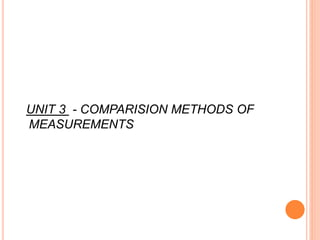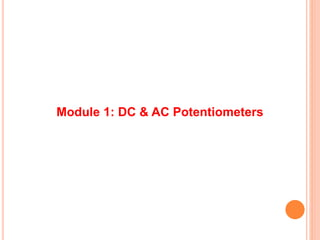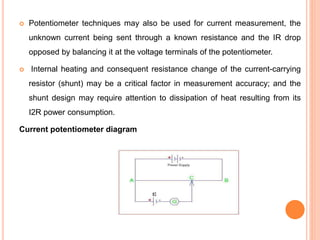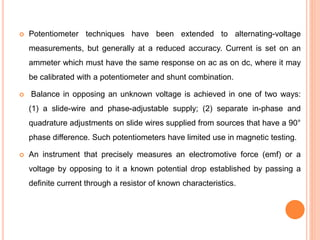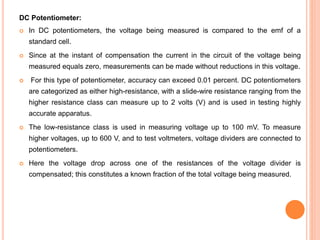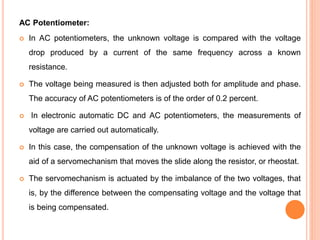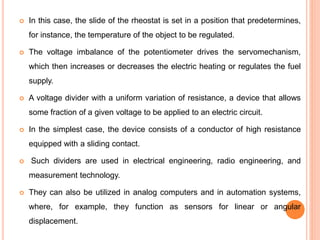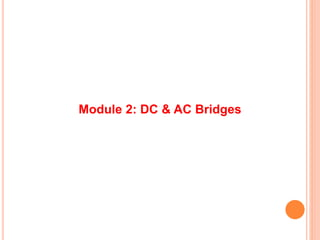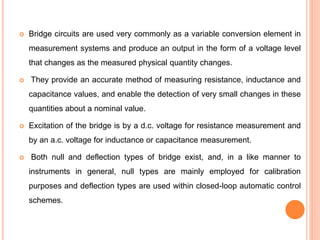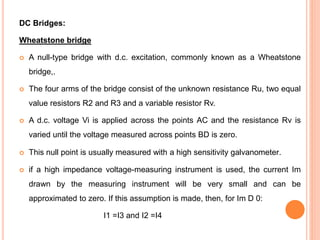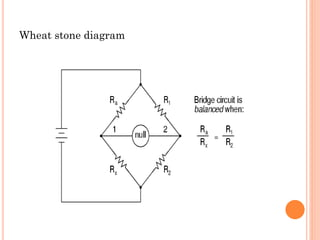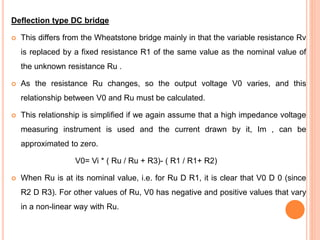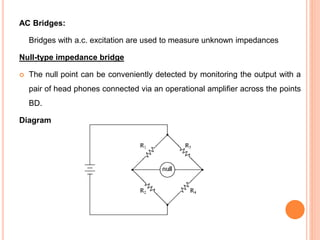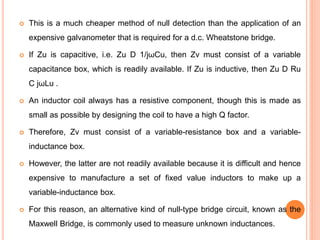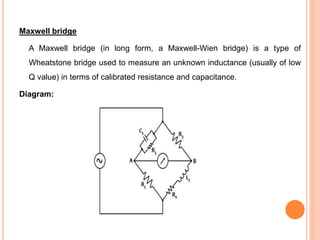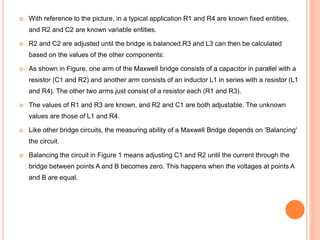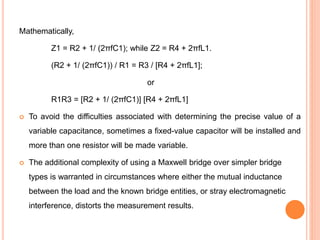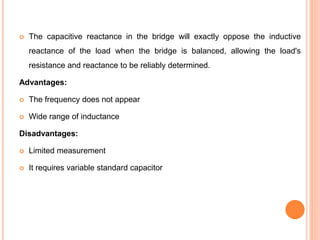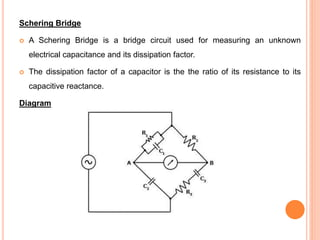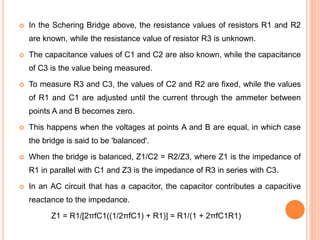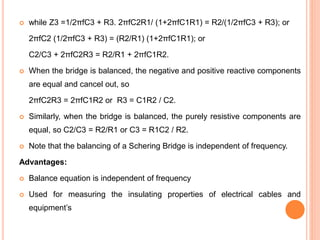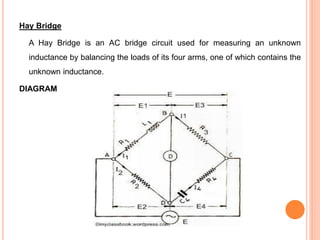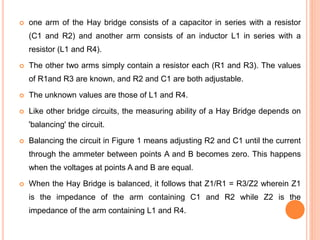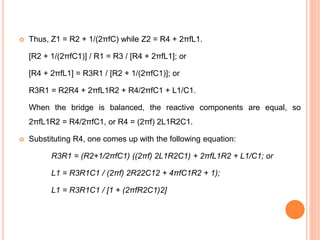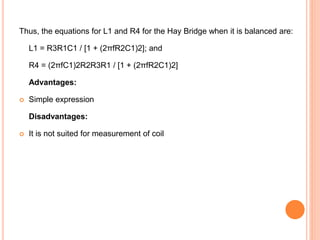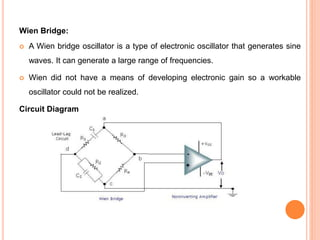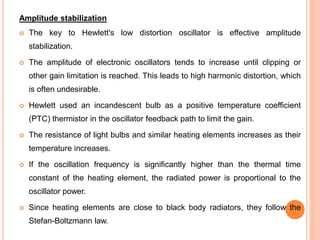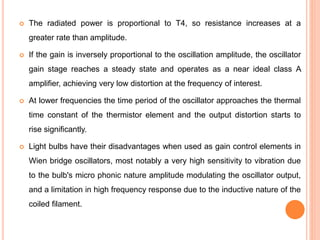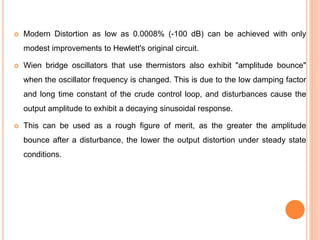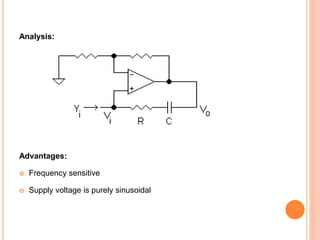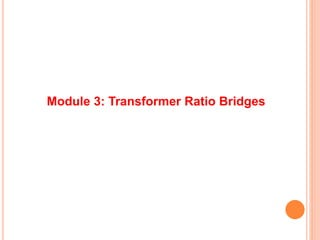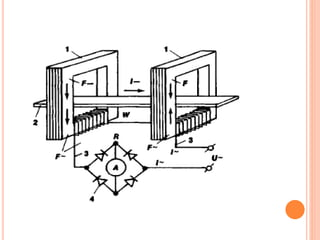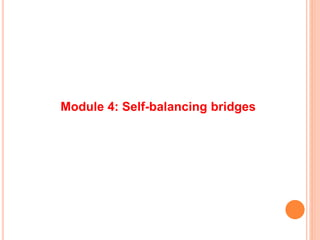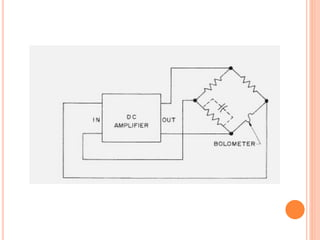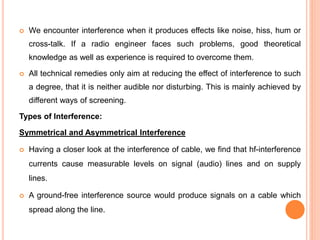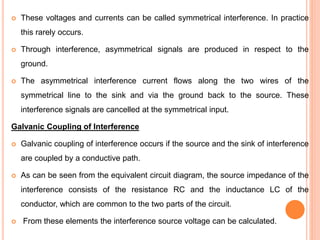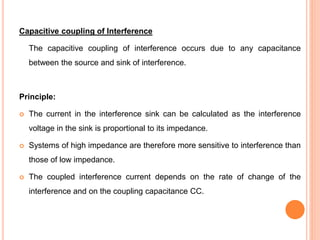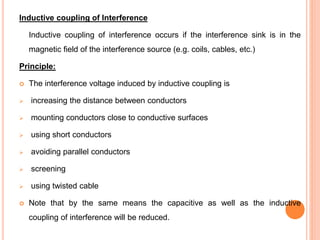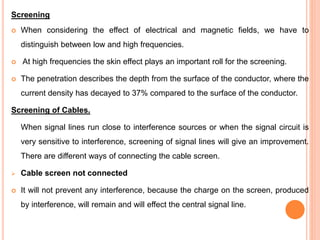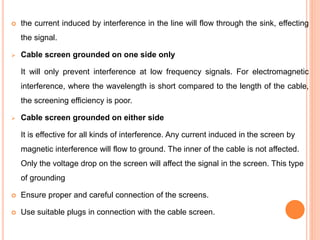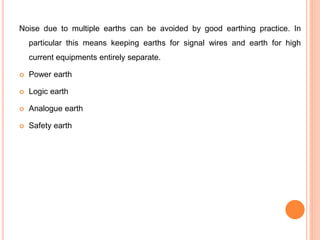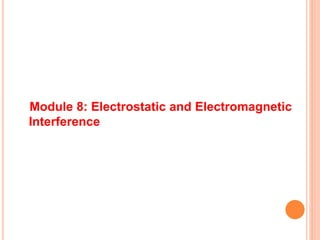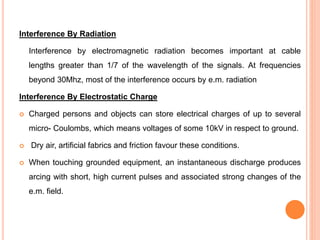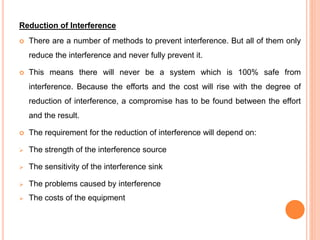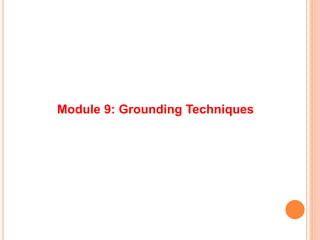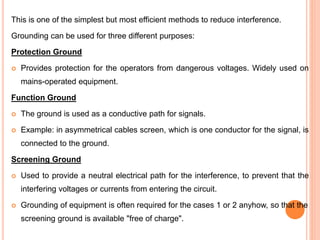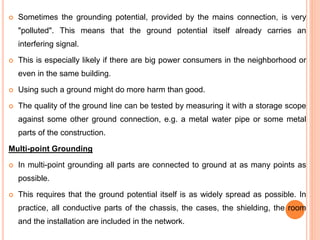1 von 50

### Comparision methods of measurements

• 1. UNIT 3 - COMPARISION METHODS OF MEASUREMENTS
• 2. Module 1: DC & AC Potentiometers
• 3.  Potentiometer techniques may also be used for current measurement, the unknown current being sent through a known resistance and the IR drop opposed by balancing it at the voltage terminals of the potentiometer.  Internal heating and consequent resistance change of the current-carrying resistor (shunt) may be a critical factor in measurement accuracy; and the shunt design may require attention to dissipation of heat resulting from its I2R power consumption. Current potentiometer diagram
• 4.  Potentiometer techniques have been extended to alternating-voltage measurements, but generally at a reduced accuracy. Current is set on an ammeter which must have the same response on ac as on dc, where it may be calibrated with a potentiometer and shunt combination.  Balance in opposing an unknown voltage is achieved in one of two ways: (1) a slide-wire and phase-adjustable supply; (2) separate in-phase and quadrature adjustments on slide wires supplied from sources that have a 90° phase difference. Such potentiometers have limited use in magnetic testing.  An instrument that precisely measures an electromotive force (emf) or a voltage by opposing to it a known potential drop established by passing a definite current through a resistor of known characteristics.
• 5. DC Potentiometer:  In DC potentiometers, the voltage being measured is compared to the emf of a standard cell.  Since at the instant of compensation the current in the circuit of the voltage being measured equals zero, measurements can be made without reductions in this voltage.  For this type of potentiometer, accuracy can exceed 0.01 percent. DC potentiometers are categorized as either high-resistance, with a slide-wire resistance ranging from the higher resistance class can measure up to 2 volts (V) and is used in testing highly accurate apparatus.  The low-resistance class is used in measuring voltage up to 100 mV. To measure higher voltages, up to 600 V, and to test voltmeters, voltage dividers are connected to potentiometers.  Here the voltage drop across one of the resistances of the voltage divider is compensated; this constitutes a known fraction of the total voltage being measured.
• 6. AC Potentiometer:  In AC potentiometers, the unknown voltage is compared with the voltage drop produced by a current of the same frequency across a known resistance.  The voltage being measured is then adjusted both for amplitude and phase. The accuracy of AC potentiometers is of the order of 0.2 percent.  In electronic automatic DC and AC potentiometers, the measurements of voltage are carried out automatically.  In this case, the compensation of the unknown voltage is achieved with the aid of a servomechanism that moves the slide along the resistor, or rheostat.  The servomechanism is actuated by the imbalance of the two voltages, that is, by the difference between the compensating voltage and the voltage that is being compensated.
• 7.  In this case, the slide of the rheostat is set in a position that predetermines, for instance, the temperature of the object to be regulated.  The voltage imbalance of the potentiometer drives the servomechanism, which then increases or decreases the electric heating or regulates the fuel supply.  A voltage divider with a uniform variation of resistance, a device that allows some fraction of a given voltage to be applied to an electric circuit.  In the simplest case, the device consists of a conductor of high resistance equipped with a sliding contact.  Such dividers are used in electrical engineering, radio engineering, and measurement technology.  They can also be utilized in analog computers and in automation systems, where, for example, they function as sensors for linear or angular displacement.
• 8. Module 2: DC & AC Bridges
• 9.  Bridge circuits are used very commonly as a variable conversion element in measurement systems and produce an output in the form of a voltage level that changes as the measured physical quantity changes.  They provide an accurate method of measuring resistance, inductance and capacitance values, and enable the detection of very small changes in these quantities about a nominal value.  Excitation of the bridge is by a d.c. voltage for resistance measurement and by an a.c. voltage for inductance or capacitance measurement.  Both null and deflection types of bridge exist, and, in a like manner to instruments in general, null types are mainly employed for calibration purposes and deflection types are used within closed-loop automatic control schemes.
• 10. DC Bridges: Wheatstone bridge  A null-type bridge with d.c. excitation, commonly known as a Wheatstone bridge,.  The four arms of the bridge consist of the unknown resistance Ru, two equal value resistors R2 and R3 and a variable resistor Rv.  A d.c. voltage Vi is applied across the points AC and the resistance Rv is varied until the voltage measured across points BD is zero.  This null point is usually measured with a high sensitivity galvanometer.  if a high impedance voltage-measuring instrument is used, the current Im drawn by the measuring instrument will be very small and can be approximated to zero. If this assumption is made, then, for Im D 0: I1 =I3 and I2 =I4
• 11. Wheat stone diagram
• 12. Deflection type DC bridge  This differs from the Wheatstone bridge mainly in that the variable resistance Rv is replaced by a fixed resistance R1 of the same value as the nominal value of the unknown resistance Ru .  As the resistance Ru changes, so the output voltage V0 varies, and this relationship between V0 and Ru must be calculated.  This relationship is simplified if we again assume that a high impedance voltage measuring instrument is used and the current drawn by it, Im , can be approximated to zero. V0= Vi * ( Ru / Ru + R3)- ( R1 / R1+ R2)  When Ru is at its nominal value, i.e. for Ru D R1, it is clear that V0 D 0 (since R2 D R3). For other values of Ru, V0 has negative and positive values that vary in a non-linear way with Ru.
• 13. AC Bridges: Bridges with a.c. excitation are used to measure unknown impedances Null-type impedance bridge  The null point can be conveniently detected by monitoring the output with a pair of head phones connected via an operational amplifier across the points BD. Diagram
• 14.  This is a much cheaper method of null detection than the application of an expensive galvanometer that is required for a d.c. Wheatstone bridge.  If Zu is capacitive, i.e. Zu D 1/jωCu, then Zv must consist of a variable capacitance box, which is readily available. If Zu is inductive, then Zu D Ru C jωLu .  An inductor coil always has a resistive component, though this is made as small as possible by designing the coil to have a high Q factor.  Therefore, Zv must consist of a variable-resistance box and a variable- inductance box.  However, the latter are not readily available because it is difficult and hence expensive to manufacture a set of fixed value inductors to make up a variable-inductance box.  For this reason, an alternative kind of null-type bridge circuit, known as the Maxwell Bridge, is commonly used to measure unknown inductances.
• 15. Maxwell bridge A Maxwell bridge (in long form, a Maxwell-Wien bridge) is a type of Wheatstone bridge used to measure an unknown inductance (usually of low Q value) in terms of calibrated resistance and capacitance. Diagram:
• 16.  With reference to the picture, in a typical application R1 and R4 are known fixed entities, and R2 and C2 are known variable entities.  R2 and C2 are adjusted until the bridge is balanced.R3 and L3 can then be calculated based on the values of the other components:  As shown in Figure, one arm of the Maxwell bridge consists of a capacitor in parallel with a resistor (C1 and R2) and another arm consists of an inductor L1 in series with a resistor (L1 and R4). The other two arms just consist of a resistor each (R1 and R3).  The values of R1 and R3 are known, and R2 and C1 are both adjustable. The unknown values are those of L1 and R4.  Like other bridge circuits, the measuring ability of a Maxwell Bridge depends on 'Balancing' the circuit.  Balancing the circuit in Figure 1 means adjusting C1 and R2 until the current through the bridge between points A and B becomes zero. This happens when the voltages at points A and B are equal.
• 17. Mathematically, Z1 = R2 + 1/ (2πfC1); while Z2 = R4 + 2πfL1. (R2 + 1/ (2πfC1)) / R1 = R3 / [R4 + 2πfL1]; or R1R3 = [R2 + 1/ (2πfC1)] [R4 + 2πfL1]  To avoid the difficulties associated with determining the precise value of a variable capacitance, sometimes a fixed-value capacitor will be installed and more than one resistor will be made variable.  The additional complexity of using a Maxwell bridge over simpler bridge types is warranted in circumstances where either the mutual inductance between the load and the known bridge entities, or stray electromagnetic interference, distorts the measurement results.
• 18.  The capacitive reactance in the bridge will exactly oppose the inductive reactance of the load when the bridge is balanced, allowing the load's resistance and reactance to be reliably determined. Advantages:  The frequency does not appear  Wide range of inductance Disadvantages:  Limited measurement  It requires variable standard capacitor
• 19. Schering Bridge  A Schering Bridge is a bridge circuit used for measuring an unknown electrical capacitance and its dissipation factor.  The dissipation factor of a capacitor is the the ratio of its resistance to its capacitive reactance. Diagram
• 20.  In the Schering Bridge above, the resistance values of resistors R1 and R2 are known, while the resistance value of resistor R3 is unknown.  The capacitance values of C1 and C2 are also known, while the capacitance of C3 is the value being measured.  To measure R3 and C3, the values of C2 and R2 are fixed, while the values of R1 and C1 are adjusted until the current through the ammeter between points A and B becomes zero.  This happens when the voltages at points A and B are equal, in which case the bridge is said to be 'balanced'.  When the bridge is balanced, Z1/C2 = R2/Z3, where Z1 is the impedance of R1 in parallel with C1 and Z3 is the impedance of R3 in series with C3.  In an AC circuit that has a capacitor, the capacitor contributes a capacitive reactance to the impedance. Z1 = R1/[2πfC1((1/2πfC1) + R1)] = R1/(1 + 2πfC1R1)
• 21.  while Z3 =1/2πfC3 + R3. 2πfC2R1/ (1+2πfC1R1) = R2/(1/2πfC3 + R3); or 2πfC2 (1/2πfC3 + R3) = (R2/R1) (1+2πfC1R1); or C2/C3 + 2πfC2R3 = R2/R1 + 2πfC1R2.  When the bridge is balanced, the negative and positive reactive components are equal and cancel out, so 2πfC2R3 = 2πfC1R2 or R3 = C1R2 / C2.  Similarly, when the bridge is balanced, the purely resistive components are equal, so C2/C3 = R2/R1 or C3 = R1C2 / R2.  Note that the balancing of a Schering Bridge is independent of frequency. Advantages:  Balance equation is independent of frequency  Used for measuring the insulating properties of electrical cables and equipment’s
• 22. Hay Bridge A Hay Bridge is an AC bridge circuit used for measuring an unknown inductance by balancing the loads of its four arms, one of which contains the unknown inductance. DIAGRAM
• 23.  one arm of the Hay bridge consists of a capacitor in series with a resistor (C1 and R2) and another arm consists of an inductor L1 in series with a resistor (L1 and R4).  The other two arms simply contain a resistor each (R1 and R3). The values of R1and R3 are known, and R2 and C1 are both adjustable.  The unknown values are those of L1 and R4.  Like other bridge circuits, the measuring ability of a Hay Bridge depends on 'balancing' the circuit.  Balancing the circuit in Figure 1 means adjusting R2 and C1 until the current through the ammeter between points A and B becomes zero. This happens when the voltages at points A and B are equal.  When the Hay Bridge is balanced, it follows that Z1/R1 = R3/Z2 wherein Z1 is the impedance of the arm containing C1 and R2 while Z2 is the impedance of the arm containing L1 and R4.
• 24.  Thus, Z1 = R2 + 1/(2πfC) while Z2 = R4 + 2πfL1. [R2 + 1/(2πfC1)] / R1 = R3 / [R4 + 2πfL1]; or [R4 + 2πfL1] = R3R1 / [R2 + 1/(2πfC1)]; or R3R1 = R2R4 + 2πfL1R2 + R4/2πfC1 + L1/C1. When the bridge is balanced, the reactive components are equal, so 2πfL1R2 = R4/2πfC1, or R4 = (2πf) 2L1R2C1.  Substituting R4, one comes up with the following equation: R3R1 = (R2+1/2πfC1) ((2πf) 2L1R2C1) + 2πfL1R2 + L1/C1; or L1 = R3R1C1 / (2πf) 2R22C12 + 4πfC1R2 + 1); L1 = R3R1C1 / [1 + (2πfR2C1)2]
• 25. Thus, the equations for L1 and R4 for the Hay Bridge when it is balanced are: L1 = R3R1C1 / [1 + (2πfR2C1)2]; and R4 = (2πfC1)2R2R3R1 / [1 + (2πfR2C1)2] Advantages:  Simple expression Disadvantages:  It is not suited for measurement of coil
• 26. Wien Bridge:  A Wien bridge oscillator is a type of electronic oscillator that generates sine waves. It can generate a large range of frequencies.  Wien did not have a means of developing electronic gain so a workable oscillator could not be realized. Circuit Diagram
• 27. Amplitude stabilization  The key to Hewlett's low distortion oscillator is effective amplitude stabilization.  The amplitude of electronic oscillators tends to increase until clipping or other gain limitation is reached. This leads to high harmonic distortion, which is often undesirable.  Hewlett used an incandescent bulb as a positive temperature coefficient (PTC) thermistor in the oscillator feedback path to limit the gain.  The resistance of light bulbs and similar heating elements increases as their temperature increases.  If the oscillation frequency is significantly higher than the thermal time constant of the heating element, the radiated power is proportional to the oscillator power.  Since heating elements are close to black body radiators, they follow the Stefan-Boltzmann law.
• 28.  The radiated power is proportional to T4, so resistance increases at a greater rate than amplitude.  If the gain is inversely proportional to the oscillation amplitude, the oscillator gain stage reaches a steady state and operates as a near ideal class A amplifier, achieving very low distortion at the frequency of interest.  At lower frequencies the time period of the oscillator approaches the thermal time constant of the thermistor element and the output distortion starts to rise significantly.  Light bulbs have their disadvantages when used as gain control elements in Wien bridge oscillators, most notably a very high sensitivity to vibration due to the bulb's micro phonic nature amplitude modulating the oscillator output, and a limitation in high frequency response due to the inductive nature of the coiled filament.
• 29.  Modern Distortion as low as 0.0008% (-100 dB) can be achieved with only modest improvements to Hewlett's original circuit.  Wien bridge oscillators that use thermistors also exhibit "amplitude bounce" when the oscillator frequency is changed. This is due to the low damping factor and long time constant of the crude control loop, and disturbances cause the output amplitude to exhibit a decaying sinusoidal response.  This can be used as a rough figure of merit, as the greater the amplitude bounce after a disturbance, the lower the output distortion under steady state conditions.
• 30. Analysis: Advantages:  Frequency sensitive  Supply voltage is purely sinusoidal
• 31. Module 3: Transformer Ratio Bridges
• 33. Module 4: Self-balancing bridges
• 35. Module 5: Interference & Screening
• 36.  We encounter interference when it produces effects like noise, hiss, hum or cross-talk. If a radio engineer faces such problems, good theoretical knowledge as well as experience is required to overcome them.  All technical remedies only aim at reducing the effect of interference to such a degree, that it is neither audible nor disturbing. This is mainly achieved by different ways of screening. Types of Interference: Symmetrical and Asymmetrical Interference  Having a closer look at the interference of cable, we find that hf-interference currents cause measurable levels on signal (audio) lines and on supply lines.  A ground-free interference source would produce signals on a cable which spread along the line.
• 37.  These voltages and currents can be called symmetrical interference. In practice this rarely occurs.  Through interference, asymmetrical signals are produced in respect to the ground.  The asymmetrical interference current flows along the two wires of the symmetrical line to the sink and via the ground back to the source. These interference signals are cancelled at the symmetrical input. Galvanic Coupling of Interference  Galvanic coupling of interference occurs if the source and the sink of interference are coupled by a conductive path.  As can be seen from the equivalent circuit diagram, the source impedance of the interference consists of the resistance RC and the inductance LC of the conductor, which are common to the two parts of the circuit.  From these elements the interference source voltage can be calculated.
• 38. Capacitive coupling of Interference The capacitive coupling of interference occurs due to any capacitance between the source and sink of interference. Principle:  The current in the interference sink can be calculated as the interference voltage in the sink is proportional to its impedance.  Systems of high impedance are therefore more sensitive to interference than those of low impedance.  The coupled interference current depends on the rate of change of the interference and on the coupling capacitance CC.
• 39. Inductive coupling of Interference Inductive coupling of interference occurs if the interference sink is in the magnetic field of the interference source (e.g. coils, cables, etc.) Principle:  The interference voltage induced by inductive coupling is  increasing the distance between conductors  mounting conductors close to conductive surfaces  using short conductors  avoiding parallel conductors  screening  using twisted cable  Note that by the same means the capacitive as well as the inductive coupling of interference will be reduced.
• 40. Screening  When considering the effect of electrical and magnetic fields, we have to distinguish between low and high frequencies.  At high frequencies the skin effect plays an important roll for the screening.  The penetration describes the depth from the surface of the conductor, where the current density has decayed to 37% compared to the surface of the conductor. Screening of Cables. When signal lines run close to interference sources or when the signal circuit is very sensitive to interference, screening of signal lines will give an improvement. There are different ways of connecting the cable screen.  Cable screen not connected  It will not prevent any interference, because the charge on the screen, produced by interference, will remain and will effect the central signal line.
• 41.  the current induced by interference in the line will flow through the sink, effecting the signal.  Cable screen grounded on one side only It will only prevent interference at low frequency signals. For electromagnetic interference, where the wavelength is short compared to the length of the cable, the screening efficiency is poor.  Cable screen grounded on either side It is effective for all kinds of interference. Any current induced in the screen by magnetic interference will flow to ground. The inner of the cable is not affected. Only the voltage drop on the screen will affect the signal in the screen. This type of grounding  Ensure proper and careful connection of the screens.  Use suitable plugs in connection with the cable screen.
• 42. Module 7: Multiple earth and Earth loops
• 43. Noise due to multiple earths can be avoided by good earthing practice. In particular this means keeping earths for signal wires and earth for high current equipments entirely separate.  Power earth  Logic earth  Analogue earth  Safety earth
• 44. Module 8: Electrostatic and Electromagnetic Interference
• 45. Interference By Radiation Interference by electromagnetic radiation becomes important at cable lengths greater than 1/7 of the wavelength of the signals. At frequencies beyond 30Mhz, most of the interference occurs by e.m. radiation Interference By Electrostatic Charge  Charged persons and objects can store electrical charges of up to several micro- Coulombs, which means voltages of some 10kV in respect to ground.  Dry air, artificial fabrics and friction favour these conditions.  When touching grounded equipment, an instantaneous discharge produces arcing with short, high current pulses and associated strong changes of the e.m. field.
• 46. Reduction of Interference  There are a number of methods to prevent interference. But all of them only reduce the interference and never fully prevent it.  This means there will never be a system which is 100% safe from interference. Because the efforts and the cost will rise with the degree of reduction of interference, a compromise has to be found between the effort and the result.  The requirement for the reduction of interference will depend on:  The strength of the interference source  The sensitivity of the interference sink  The problems caused by interference  The costs of the equipment
• 47. Module 9: Grounding Techniques
• 48. This is one of the simplest but most efficient methods to reduce interference. Grounding can be used for three different purposes: Protection Ground  Provides protection for the operators from dangerous voltages. Widely used on mains-operated equipment. Function Ground  The ground is used as a conductive path for signals.  Example: in asymmetrical cables screen, which is one conductor for the signal, is connected to the ground. Screening Ground  Used to provide a neutral electrical path for the interference, to prevent that the interfering voltages or currents from entering the circuit.  Grounding of equipment is often required for the cases 1 or 2 anyhow, so that the screening ground is available "free of charge".
• 49.  Sometimes the grounding potential, provided by the mains connection, is very "polluted". This means that the ground potential itself already carries an interfering signal.  This is especially likely if there are big power consumers in the neighborhood or even in the same building.  Using such a ground might do more harm than good.  The quality of the ground line can be tested by measuring it with a storage scope against some other ground connection, e.g. a metal water pipe or some metal parts of the construction. Multi-point Grounding  In multi-point grounding all parts are connected to ground at as many points as possible.  This requires that the ground potential itself is as widely spread as possible. In practice, all conductive parts of the chassis, the cases, the shielding, the room and the installation are included in the network.
• 50. Thank you